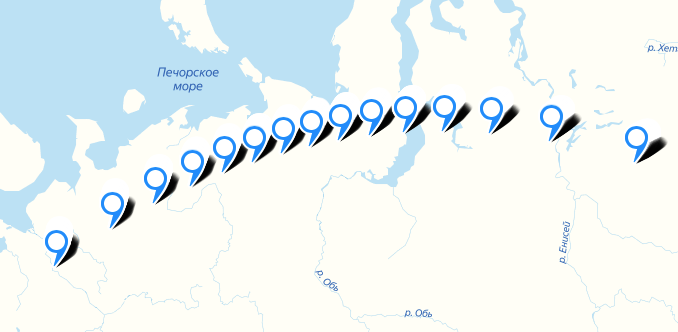# Wide angle sensor

Hi all!

I’m trying to rectify images from Meteor-M 2. It has wide angle camera: 110.5 degrees. When I’m doing this:

``````	int width = 1568;
List<Vector3D> rawDirs = new ArrayList<Vector3D>();
for (int i = 0; i < width; i++) {
}
``````

I’m getting:

``````	org.orekit.rugged.errors.RuggedException: line-of-sight does not reach ground
``````

I guess this exception might be caused by the wide angle camera.

Can you help me simulate camera with wide angle?

The Vector3D constructor takes x, y and z as parameters, so your coordinates seem weird to me as the y coordinate seems to be an angle whereas the first and the x is set to 0 and y set to 1.0.
So if your camera lines of sights are in the (YZ) plane, with the +Z axis as the middle point, the construction loop should be:

``````final int width = 1568;
final double delta = FastMath.toRadians(110.5) / width;
final List<Vector3D> rawDirs = new ArrayList<>();
for (int i = 0; i < width; i++) {
final Sincos sc = FastMath.sinCos((i - width / 2) * delta);
}
``````

Beware that with an even width, there is not pixel exactly aligned with the +Z direction, you may need to adjust the formula above to your accurate geometry.

There is a two arguments constructor that takes angles (azimuth, i.e angle around Z with a 2π range and elevation above XY plane between -π/2 and +π/2), but it would be weird to use for a line in the (YZ) plane.

1 Like

Thank you so much!

It works. At least I’m seeing bigger distances between pixels on the edges.